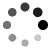[原创]【计算几何各种知识点总结】[不定期补充]

2016-04-10 19:26:51 Tabris_ 阅读数：3490

https://blog.csdn.net/qq_33184171/article/details/51114511

#计算几何模板总结 ：

## 精度控制

const double PI = acos(-1.0);
const double EPS = 1e-8;

eg：8(double)=7.9999999999…;
8(int)=8;
int(8(double))=7; !!!

## 杂点

### 精度问题

1.在用1e-n判断相等的时候不是n越大越好 要选择合适的 ，一般为6/8/9/10
2.判断两条线段距离大小关系的时候有平方倍进行比较。
3.未完待续。。。

## 点与其他图形的位置关系

### 点与直线的位置关系

1.点在直线上2.点不在直线上### 点与线段的关系

1.点在线段上
2.点不在线段上

1.点在线段所在的直线上。
2.点在以线段为对角线的矩形内。c.x> min(a.x,b.x);
c.x< max(a.x,b.x);
c.y> min(a.y,b.y);
c.y< max(a.y,b.y);

### 点与三角形的内外S△dab+S△dbc+S△dac是大于S△abc的

Ps：这里求面积运用的为叉乘,$\overrightarrow{ab} \times\overrightarrow{ac}$就是 延伸出去的平行四边形的面积
$S△_{abc}=\dfrac{\overrightarrow{ab} \times\overrightarrow{ac} }{2}$

## 线段相交

### 判断两线段是否相交

1.相离2.相交3.重合4.平行Ps：如果不了解叉乘的话必须先了解下叉乘，但我相信能做到求线段相交的人一定对叉乘有了全方位的了解$(\overrightarrow{CD} \times\overrightarrow{DA})\cdot(\overrightarrow{CD} \times\overrightarrow{DB})<0;$
$(\overrightarrow{AB} \times\overrightarrow{BD})\cdot(\overrightarrow{AB} \times\overrightarrow{BC})<0;$

(这部分证明并没有什么意义，大家想想即可明白，在此就不赘述了，支架以代码形式给出)

max(s1.x,e1.x) < min(s2.x,e2.x)
max(s2.x,e2.x) < min(s1.x,e1.x)
max(s1.y,e1.y) < min(s2.y,e2.y)
max(s2.y,e2.y) < min(s1.y,e1.y)

======

## 求两圆相交面积

1.内含 2.内切 3.相交 4.外切 5.外离

Ps：如果有疑问可以自己画一画图 就会明白了

1.外离2.内含3.相交S扇形abc1-S△abc1+S扇形abc2-S△abc2<=>
S扇形abc1+S扇形abc2-S□ac1bc2;

$ang1 = acos(\dfrac{r1 * r1 + d * d - r2 * r2}{2.0*r1*d})$
$ang2 = acos(\dfrac{r2 * r2 + d * d - r1 * r1}{2.0r2d})S扇形_{abc1}=ang1 * r1 * r1S扇形_{abc2}=ang2 * r2 * r2S□ac1bc2=d * r1 * sin(ang1)$

$S = ang1 * r1 * r1+ang2 * r2 * r2 - d * r1 * sin(ang1)；$

======